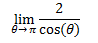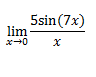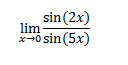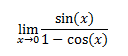Clutch Prep is now a part of Pearson
Ch.4: Limits (Part 1)WorksheetSee all chapters

# Limits of Trigonometric Functions

See all sections
Sections
Introduction to Limits
Limits at Infinity
Computing Limits
Limits of Trigonometric Functions

Concept #1

Concept #2: Intro

Practice: Evaluate the following limitPractice: Evaluate the following limitPractice: Evaluate the following limitPractice: Evaluate the following limit